# Exploratory Data Analysis in Python

## What is Exploratory Data Analysis (EDA) ?

EDA is a phenomenon under data analysis used for gaining a better understanding of data aspects like:
– main features of data
– variables and relationships that hold between them
– identifying which variables are important for our problem
We shall look at various exploratory data analysis methods like:

• Descriptive Statistics, which is a way of giving a brief overview of the dataset we are dealing with, including some measures and features of the sample
• Grouping data [Basic grouping with group by]
• ANOVA, Analysis Of Variance, which is a computational method to divide variations in an observations set into different components.
• Correlation and correlation methods

The dataset we’ll be using is chile voting dataset, which you can import in python as:

 `import` `pandas as pd ` `Df ``=` `pd.read_csv(``"https://vincentarelbundock.github.io / Rdatasets / csv / car / Chile.csv"``) `

## Descriptive Statistics

Descriptive statistics is a helpful way to understand characteristics of your data and to get a quick summary of it. Pandas in python provide an interesting method describe(). The describe function applies basic statistical computations on the dataset like extreme values, count of data points standard deviation etc. Any missing value or NaN value is automatically skipped. describe() function gives a good picture of distribution of data.

 `DF.describe() `

Here’s the output you’ll get on running above code: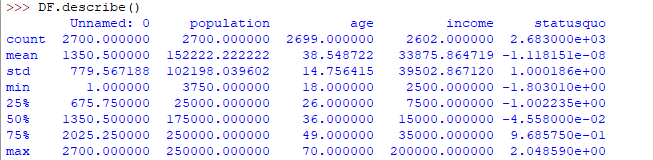Another useful method if value_counts() which can get count of each category in a categorical attributed series of values. For an instance suppose you are dealing with a dataset of customers who are divided as youth, medium and old categories under column name age and your dataframe is “DF”. You can run this statement to know how many people fall in respective categories. In our data set example education column can be used

 `DF[``"education"``].value_counts() `

The output of the above code will be: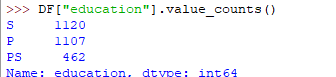One more useful tool is boxplot which you can use through matplotlib module. Boxplot is a pictorial representation of distribution of data which shows extreme values, median and quartiles. We can easily figure out outliers by using boxplots. Now consider the dataset we’ve been dealing with again and lets draw a boxplot on attribute population

 `import` `pandas as pd ` `import` `matplotlib.pyplot as plt ` `DF ``=` `pd.read_csv(``"https://raw.githubusercontent.com / fivethirtyeight / data / master / airline-safety / airline-safety.csv"``) ` `y ``=` `list``(DF.population) ` `plt.boxplot(y) ` `plt.show() `

The output plot would look like this with spotting out outliers: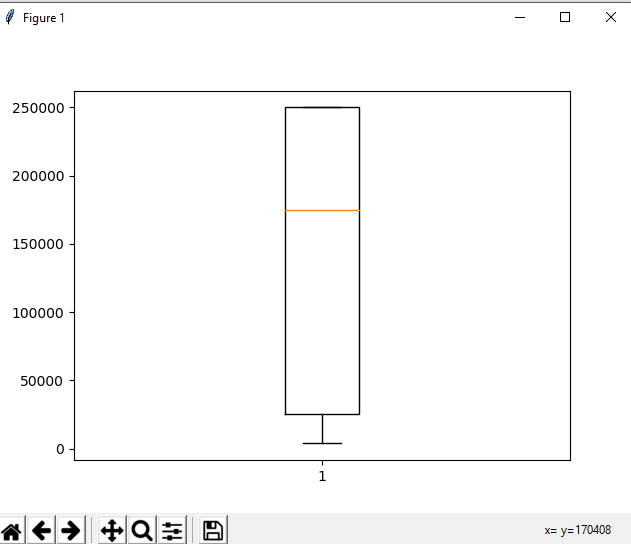## Grouping data

Group by is an interesting measure available in pandas which can help us figure out effect of different categorical attributes on other data variables. Let’s see an example on the same dataset where we want to figure out affect of people’s age and education on the voting dataset.

 `DF.groupby([``'education'``, ``'vote'``]).mean() `

The output would be somewhat like this: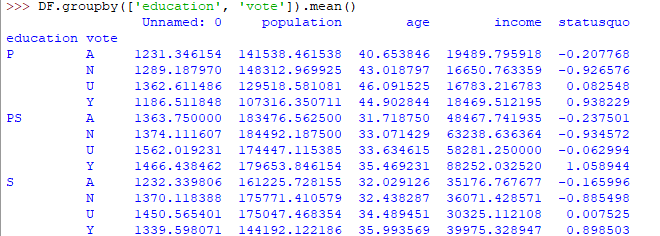If this group by output table is less understandable further analysts use pivot tables and heat maps for visualization on them.

## ANOVA

ANOVA stands for Analysis of Variance. It is performed to figure out the relation between the different group of categorical data.
Under ANOVA we have two measures as result:
– F-testscore : which shows the variaton of groups mean over variation
– p-value: it shows the importance of the result
This can be performed using python module scipy method name f_oneway()
Syntax:

``````
import scipy.stats as st
st.f_oneway(sample1, sample2, ..)
``````

These samples are sample measurements for each group.
As a conclusion, we can say that there is a strong correlation between other variables and a categorical variable if the ANOVA test gives us a large F-test value and a small p-value.

## Correlation and Correlation computation

Correlation is a simple relationship between two variables in a context such that one variable affects the other. Correlation is different from act of causing. One way to calculate correlation among variables is to find Pearson correlation. Here we find two parameters namely, Pearson coefficient and p-value. We can say there is a strong correlation between two variables when Pearson correlation coefficient is close to either 1 or -1 and the p-value is less than 0.0001.
Scipy module also provides a method to perform pearson correlation analysis, syntax:

``````import scipy.stats as st
st.pearsonr(sample1, sample2)
``````

Here samples are the attributes you want to compare.
This is a brief overview of EDA in python, we can do lots more! Happy digging!

My Personal Notes arrow_drop_up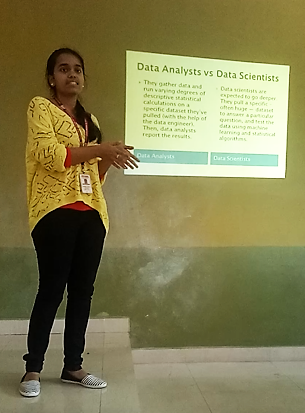Check out this Author's contributed articles.

If you like GeeksforGeeks and would like to contribute, you can also write an article using contribute.geeksforgeeks.org or mail your article to contribute@geeksforgeeks.org. See your article appearing on the GeeksforGeeks main page and help other Geeks.

Please Improve this article if you find anything incorrect by clicking on the "Improve Article" button below.

Article Tags :

7

Please write to us at contribute@geeksforgeeks.org to report any issue with the above content.